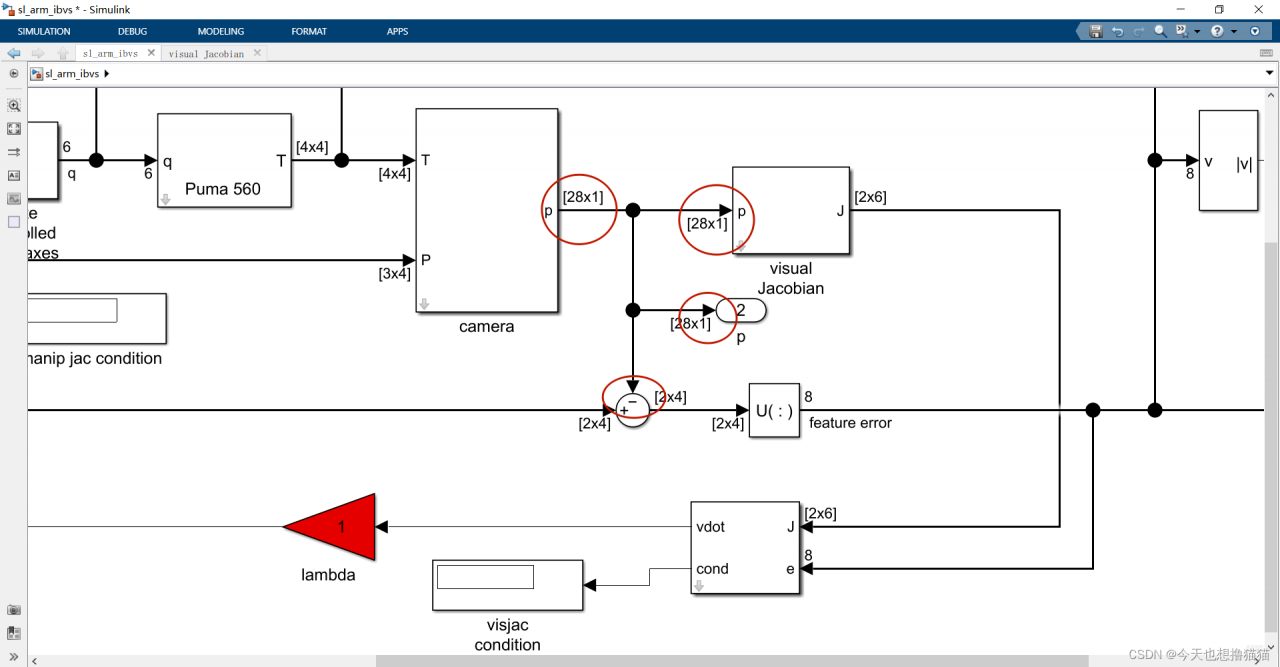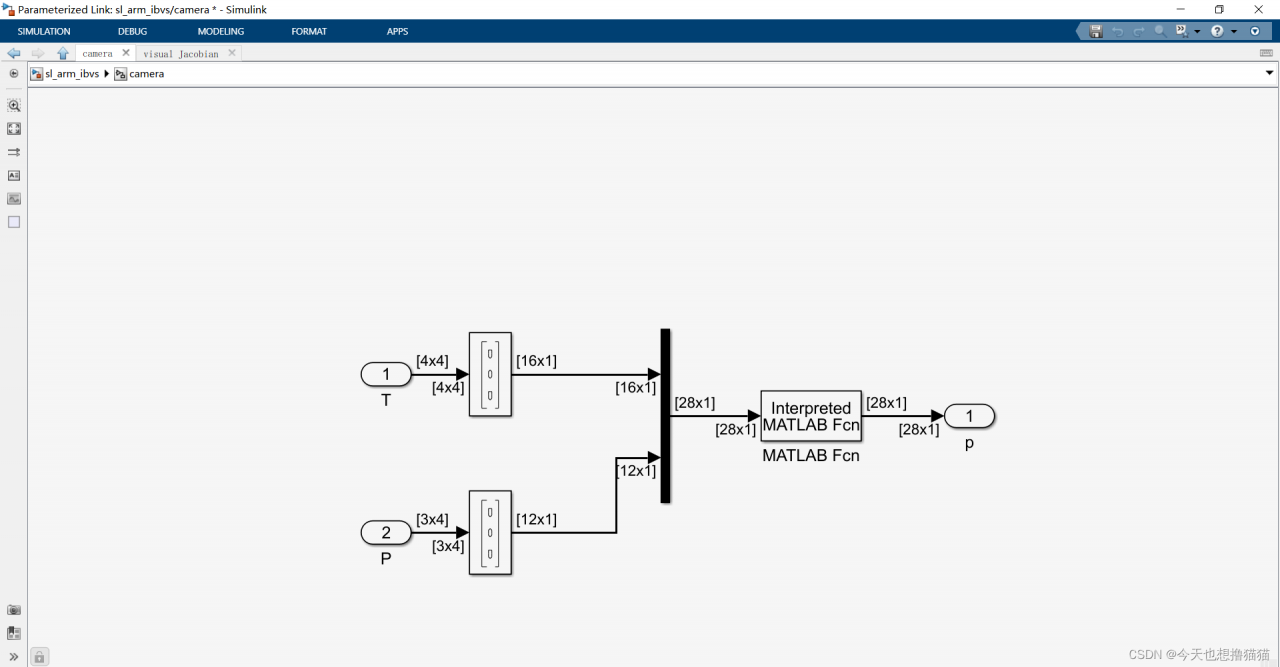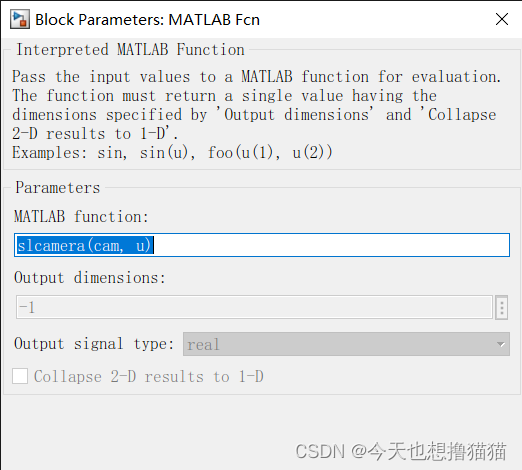# [Solved] matlab Error: Error in port widths or dimensions. ‘Output Port 1‘ of ‘sl_arm_ibvs/camera‘

When running the Manipulator Simulation in MATLAB visual servo toolbox, the following problems are encountered: error in port widths or dimensions. ‘ Output Port 1’ of ‘sl_ arm_ ibvs/camera’ is a [28×1] matrix.

The problem is caused by the wrong input vector dimension at the red circle dimension in the figure below.Here the p for the camera pixel coordinate system under the coordinate value, should be 2 * 4 vectors.

Solution: The new version of Machine Vision Toolbox for MATLAB toolbox lacks the module “slcamera.m”, open the camera module in simulate.Double click the MATLAB FCN module, and you will be prompted that “slcamera” cannot be found. Select new:Type the following code:

``````function p = slcamera(cam, u)
%if all(u == 0)
if (u(1:16,:)==0)
% Simulink is probing for the dimensions
np = (length(u)-16)/3;
p = zeros(2, np);
else
p = zeros(2, 4);
P = reshape(u(17:end), 3, []);
Tcam = reshape(u(1:16), 4, 4);
p = cam.plot(P, 'pose', Tcam, 'drawnow');

end``````

Run simulate again, you can see the error report and disappear, then you should encounter the following error.

Error in ‘sl_arm_ibvs/camera/MATLAB Fcn’. Evaluation of expression resulted in an invalid output.
Only finite double vector or matrix outputs are supported

I am also working on a solution for this error, so please stay tuned.